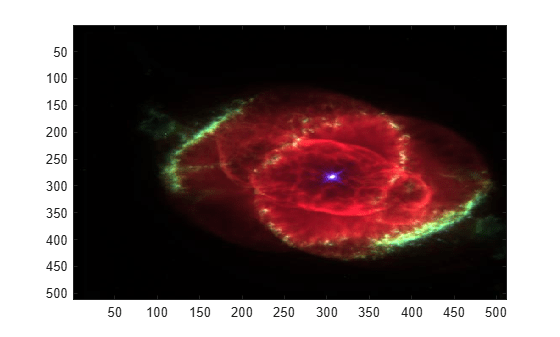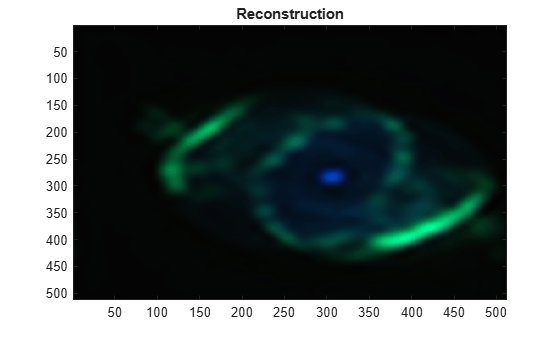# iswt2

Inverse discrete stationary 2-D wavelet transform

## Syntax

``X = iswt2(swc,wname)``
``X = iswt2(swc,LoR,HiR)``
``X = iswt2(A,H,V,D,wname)``
``X = iswt2(A,H,V,D,LoR,HiR)``

## Description

````X = iswt2(swc,wname)` returns the inverse discrete stationary 2-D wavelet transform of the wavelet decomposition `swc` using the wavelet `wname`. The decomposition `swc` is the output of `swt2`. Note`swt2` uses double-precision arithmetic internally and returns double-precision coefficient matrices. `swt2` warns if there is a loss of precision when converting to double. ```
````X = iswt2(swc,LoR,HiR)` uses the specified lowpass and highpass wavelet reconstruction filters `LoR` and `HiR`, respectively.```

example

````X = iswt2(A,H,V,D,wname)` uses the approximation coefficients array `A` and detail coefficient arrays `H`, `V`, and `D`. The arrays `H`, `V`, and `D` contain the horizontal, vertical, and diagonal detail coefficients, respectively. The arrays are the output of `swt2`.If the decomposition `swc` or the coefficient arrays `A`, `H`, `V`, and `D` were generated from a multilevel decomposition of a 2-D matrix, the syntax ```X = iswt2(A(:,:,end),H,V,D,wname)``` reconstructs the 2-D matrix.If the decomposition `swc` or the coefficient arrays `A`, `H`, `V`, and `D` were generated from a single-level decomposition of a 3-D array, the syntax ```X = iswt2(A(:,:,1,:),H,V,D,wname)``` reconstructs the 3-D array.```

example

````X = iswt2(A,H,V,D,LoR,HiR)` uses the lowpass and highpass wavelet reconstruction filters `LoR` and `HiR`, respectively.If the decomposition `swc` or the coefficient arrays `A`, `H`, `V`, and `D` were generated from a multilevel decomposition of a 2-D matrix, the syntax ```X = iswt2(A(:,:,end),H,V,D,LoR,HiR)``` reconstructs the 2-D matrix.If the decomposition `swc` or the coefficient arrays `A`, `H`, `V`, and `D` were generated from a single-level decomposition of a 3-D array, the syntax ```X = iswt2(A(:,:,1,:),H,V,D,LoR,HiR)``` reconstructs the 3-D array.```

## Examples

collapse all

Show perfect reconstruction using `swt2` and `iswt2` with an orthogonal wavelet.

```load woman [Lo_D,Hi_D,Lo_R,Hi_R] = wfilters('db6'); [ca,chd,cvd,cdd] = swt2(X,3,Lo_D,Hi_D); recon = iswt2(ca,chd,cvd,cdd,Lo_R,Hi_R); norm(X-recon)```
```ans = 1.0126e-08 ```

This example shows how to reconstruct an RGB image from a multilevel stationary wavelet decomposition using approximation and detail coefficient arrays.

Load an RGB image. An RGB image is also referred to as a truecolor image. The image is a 3-D array of type `uint8`. Since `swt2` requires that the first and second dimensions both be divisible by a power of 2, extract a portion of the image and view it.

```imdata = imread('ngc6543a.jpg'); x = imdata(1:512,1:512,:); image(x)```Obtain the level 4 stationary wavelet decomposition of the image using the `db4` wavelet. Return the approximation coefficients and horizontal, vertical, and detail coefficients as separate arrays.

`[a,h,v,d] = swt2(x,4,'db4');`

Reconstruct an image using the green and blue components of the approximation coefficients. Display the reconstruction.

```a2 = zeros(size(a)); a2(:,:,2:3,4)=a(:,:,2:3,4); xrec = iswt2(a2,0*h,0*v,0*d,'db4'); xrec2 = (xrec-min(xrec(:)))/(max(xrec(:))-min(xrec(:))); image(xrec2) title('Reconstruction')```## Input Arguments

collapse all

Stationary wavelet decomposition, specified as a 3-D or 4-D array. The decomposition contains the approximation and detail coefficients of the 2-D stationary wavelet transform (SWT). The stationary wavelet decomposition is the output of `swt2`.

Data Types: `double`

Analyzing wavelet, specified as a character vector or string scalar. `iswt2` supports only Type 1 (orthogonal) or Type 2 (biorthogonal) wavelets. See `wfilters` for a list of orthogonal and biorthogonal wavelets. The specified wavelet must be the same wavelet used to obtain the approximation and detail coefficients.

Wavelet reconstruction filters, specified as a pair of even-length real-valued vectors. `LoR` is the lowpass reconstruction filter, and `HiR` is the highpass reconstruction filter. The lengths of `LoR` and `HiR` must be equal. See `wfilters` for additional information.

Approximation coefficients, specified as a multidimensional array. The array is the output of `swt2`.

Data Types: `double`

Detail coefficients, specified as multidimensional arrays of equal size. `H`, `V`, and `D` contain the horizontal, vertical, and diagonal detail coefficients, respectively. The arrays are the output of `swt2`.

Data Types: `double`

## Output Arguments

collapse all

Reconstruction, returned as a 2-D matrix or 3-D array.

If `swc` or (`A`,`H,V,D`) are obtained from an indexed image analysis or a truecolor (RGB) image analysis, then `X` is an m-by-n matrix or an m-by-n-by-3 array, respectively.

 Nason, G. P., and B. W. Silverman. “The Stationary Wavelet Transform and Some Statistical Applications.” In Wavelets and Statistics, edited by Anestis Antoniadis and Georges Oppenheim, 103:281–99. New York, NY: Springer New York, 1995. https://doi.org/10.1007/978-1-4612-2544-7_17.

 Coifman, R. R., and D. L. Donoho. “Translation-Invariant De-Noising.” In Wavelets and Statistics, edited by Anestis Antoniadis and Georges Oppenheim, 103:125–50. New York, NY: Springer New York, 1995. https://doi.org/10.1007/978-1-4612-2544-7_9.

 Pesquet, J.-C., H. Krim, and H. Carfantan. “Time-Invariant Orthonormal Wavelet Representations.” IEEE Transactions on Signal Processing 44, no. 8 (August 1996): 1964–70. https://doi.org/10.1109/78.533717.